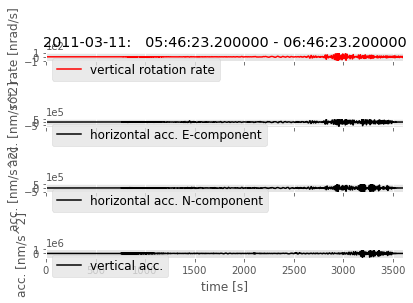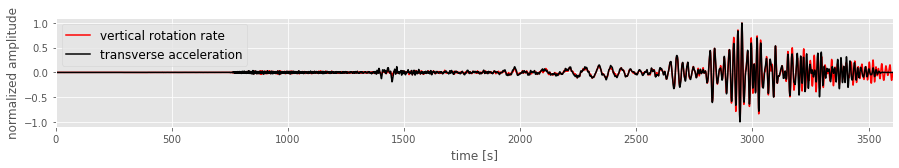Rotational Seismology

Seismo-Live: http://seismo-live.org

#### How do I download ring laser data? What pre-processing do I need to perform? ... and how can I do this using Obspy?In :
%matplotlib inline
import matplotlib.pyplot as plt
plt.style.use('ggplot')
plt.rcParams['figure.figsize'] = 12, 8


### Pick events from catalog to define start- and endtimes of traces¶

The first step to compare waveform data of a ring laser and a collocated seismometer is to choose which time period we want to look at.
Usually, we want to observe Love waves generated by earthquake events, so we need to pick an event from a earthquake catalog
which is done using the FDSN client.

The FDSN client fetches an event catalog from a data center - in this case IRIS - which can be filtered by different criteria, such as:

• magnitude
• longitude & latitude
• depth
• starttime & endtime
• catalog

The catalog we use here is the Global CMT catalog which contains moment magnitude picked events.

In :
from obspy.clients.fdsn import Client as fdsnClient
from obspy.core import UTCDateTime

c_fdsn = fdsnClient('IRIS')
cat = c_fdsn.get_events(minmagnitude=9.0, starttime=UTCDateTime(2011,1,1), endtime=UTCDateTime(2012,1,1))
event = cat
print(cat)
start = event.origins.time
end = start + 3600

1 Event(s) in Catalog:
2011-03-11T05:46:23.200000Z | +38.296, +142.498 | 9.1 MW


Once we selected an event and obtained the earthquake's origin time, we can download the waveform data using the Arclink client, which is a distributed data request protocol that can be used to access archived waveform data (for more information see Obspy's Arclink documentation)

For each requested waveform component we need to specify its unique identifier, consisting of:

• network
• station
• location
• channel
• starttime & endtime

In order to simplify the subsequent handling, we unite the three seismometer components [N,E,Z] (= traces) to a single stream object.

In :
from obspy.core.stream import Stream

c_rot = fdsnClient('http://erde.geophysik.uni-muenchen.de')  # rotational data source
c_bb  = fdsnClient('BGR')  # broadband data source

RLAS = c_rot.get_waveforms(network='BW', station='RLAS', location='', channel='BJZ', starttime=start, endtime=end)
BHE = c_bb.get_waveforms(network='GR', station='WET', location='', channel='BHE', starttime=start, endtime=end)
BHN = c_bb.get_waveforms(network='GR', station='WET', location='', channel='BHN', starttime=start, endtime=end)
BHZ = c_bb.get_waveforms(network='GR', station='WET', location='', channel='BHZ', starttime=start, endtime=end)
AC = Stream(traces=[BHE,BHN,BHZ])

print(RLAS)
print(AC)

1 Trace(s) in Stream:
BW.RLAS..BJZ | 2011-03-11T05:46:00.300899Z - 2011-03-11T06:46:30.450899Z | 20.0 Hz, 72604 samples
3 Trace(s) in Stream:
GR.WET..BHE | 2011-03-11T05:46:13.974999Z - 2011-03-11T06:46:25.574999Z | 20.0 Hz, 72233 samples
GR.WET..BHN | 2011-03-11T05:46:22.574999Z - 2011-03-11T06:46:31.024999Z | 20.0 Hz, 72170 samples
GR.WET..BHZ | 2011-03-11T05:46:06.324999Z - 2011-03-11T06:46:30.274999Z | 20.0 Hz, 72480 samples


### Remove the instrument responses from the recordings + convert units¶

We want to deal with meaningful SI-units, so we need to correct the waveforms.
To convert ring laser's vertical rotation rate to [nrad/s] units, we can simply us a conversion factor:

In :
RLAS.detrend(type='linear')
RLAS.data = RLAS.data * 1/6.3191 * 1e-3


In order to remove the seismometer's response using and convert the velocity recordings to acceleration [nm/s²],
Obspy's simulate function is used.
It removes the sensitivity and converts to acceleration in one step employing poles & zeros in this case.

In :
AC.detrend(type='linear')
AC.taper(max_percentage=0.05)

paz_sts2 = {'poles': [(-0.0367429 + 0.036754j), (-0.0367429 - 0.036754j)],
'sensitivity': 0.944019640,
'zeros': [0j],
'gain': 1.0}

AC.simulate(paz_remove=paz_sts2, remove_sensitivity=True)

Out:
3 Trace(s) in Stream:
GR.WET..BHE | 2011-03-11T05:46:13.974999Z - 2011-03-11T06:46:25.574999Z | 20.0 Hz, 72233 samples
GR.WET..BHN | 2011-03-11T05:46:22.574999Z - 2011-03-11T06:46:31.024999Z | 20.0 Hz, 72170 samples
GR.WET..BHZ | 2011-03-11T05:46:06.324999Z - 2011-03-11T06:46:30.274999Z | 20.0 Hz, 72480 samples

The resulting traces are trimmed to make sure that start- and endtimes match for all waveforms:

In :
startaim = max([tr.stats.starttime for tr in (AC + RLAS)])
endtaim = min([tr.stats.endtime for tr in (AC + RLAS)])

AC.trim(startaim, endtaim, nearest_sample=True)
RLAS.trim(startaim, endtaim, nearest_sample=True)

Out:
1 Trace(s) in Stream:
BW.RLAS..BJZ | 2011-03-11T05:46:22.550899Z - 2011-03-11T06:46:25.550899Z | 20.0 Hz, 72061 samples

### Plot the resulting traces¶

The single traces/components are plotted and compared.

In :
import matplotlib.pyplot as plt
fig, (ax1,ax2,ax3,ax4) = plt.subplots(4, sharex=True)

ax1.set_title(str(start.date) + ':   ' + str(start.time) + " - " + str(end.time))
ax1.plot(RLAS.times(), RLAS,'r',label='vertical rotation rate')
ax2.plot(AC.times(),AC,'k',label='horizontal acc. E-component')
ax2.set_ylabel('acc. [nm/s^2]')
ax3.plot(AC.times(),AC,'k',label='horizontal acc. N-component')
ax3.set_ylabel('acc. [nm/s^2]')
ax4.plot(AC.times(),AC,'k',label='vertical acc.')
ax4.set_ylabel('acc. [nm/s^2]')
ax4.set_xlabel('time [s]')

for ax in [ax1,ax2,ax3,ax4]:
ax.legend(loc=2, prop={"size":12})
ax.yaxis.major.formatter.set_powerlimits((-1,2))
ax.set_xlim(0,max(AC.times()))

fig.tight_layout()
plt.show()### Resample, Filter and Rotate¶

Resample seismograms using decimate in order to reduce the size of the arrays (speeds up processing). The seismograms are high-cut and low-cut filtered, depending on the frequency range of interest and the resolution of the instruments.

In :
RLAS.decimate(factor=4)
AC.decimate(factor=4)
high_cut = 1.0
low_cut = 0.005

RLAS.filter('bandpass', freqmax=high_cut, freqmin=low_cut, corners=2, zerophase=True)
AC.filter('bandpass', freqmax=high_cut, freqmin=low_cut, corners=2, zerophase=True)

Out:
3 Trace(s) in Stream:
GR.WET..BHE | 2011-03-11T05:46:22.574999Z - 2011-03-11T06:46:25.574999Z | 5.0 Hz, 18016 samples
GR.WET..BHN | 2011-03-11T05:46:22.574999Z - 2011-03-11T06:46:25.574999Z | 5.0 Hz, 18016 samples
GR.WET..BHZ | 2011-03-11T05:46:22.574999Z - 2011-03-11T06:46:25.574999Z | 5.0 Hz, 18016 samples

In order to align the seismometer recordings with the event direction, we need to rotate the horizontal components
of the acceleration to transverse and radial.

We can determine the theoretical rotation/direction angle (= backazimuth) from station and event location using gps2dist_azimuth.
This function also yields the epicentral distance and the azimuth angle.

In :
from obspy.geodetics.base import gps2dist_azimuth

# event location from event info
source_latitude = event.origins.latitude
source_longitude = event.origins.longitude

# station location (Wettzell)
station_latitude = 49.144001
station_longitude = 12.8782

# theoretical backazimuth and distance
baz = gps2dist_azimuth(source_latitude, source_longitude, station_latitude, station_longitude)

print('Epicentral distance [m]: ', baz)
print('Theoretical azimuth [deg]: ', baz)
print('Theoretical backazimuth [deg]: ', baz)

Epicentral distance [m]:  9127350.828883186
Theoretical azimuth [deg]:  329.40798115843046
Theoretical backazimuth [deg]:  37.602787067188785


We now rotate the E-N component seismometer recordings to radial  and transverse  acceleration using the theoretical BAz.

In a last step the normalized transverse acceleration is plotted together with **vertical rotation rate**.

In :
from obspy.signal.rotate import rotate_ne_rt
import numpy as np

# rotate
AC.rotate(method='NE->RT',back_azimuth=baz)
plt.figure(figsize=(15,2))

ax = plt.subplot(111)
ax.plot(RLAS.times(), RLAS.data/np.max(np.abs(RLAS.data)), 'r', label='vertical rotation rate')
ax.plot(AC.times(), AC.data/np.max(np.abs(AC.data)), 'k', label='transverse acceleration')
ax.legend(loc=2, prop={"size":12})
ax.set_xlabel('time [s]')
ax.set_ylabel('normalized amplitude')
ax.set_xlim(0,max(RLAS.times()))

plt.show()Note: the vertical rotation rate and transverse acceleration are in phase!